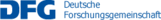Institute for applied mathematics

## C01 – Wasserstein geometry, Ricci curvature and geometric analysis

In the last decade, the concept of optimal transport has led to important breakthroughs in our understanding of heat flow and Ricci curvature on manifolds and metric measure spaces with plenty of impressive applications, among others to geometric and functional inequalities. In two independent contributions Lott and Villani [LV09] and Sturm [Stu06a*, Stu06b*] introduced generalized lower Ricci bounds for metric measure spaces (X, d, m), based on convexity properties of entropy-like functionals on the Wasserstein space $\mathcal P_2(X)$ of probability measures on X.

On metric measure spaces satisfying such bounds the heat flow can be defined equally as the gradient flow of the energy in L2-space or as the gradient flow of the entropy in Wasserstein space. The generalized Ricci bounds imply a scale-invariant local Poincaré inequality and a doubling property and thus allow to apply techniques and results of a “rough analysis” in the sense of De Giorgi-Moser-Nash.

However, these generalized Ricci bounds should allow to deduce much more precise estimates. Indeed, the curvature bounds in the sense of Lott-Sturm-Villani imply a large number of geometric and functional analytic inequalities with sharp constants, among them the Brunn-Minkowski inequality, the Bishop-Gromov volume growth estimate, the Bonnet-Myers diameter bound, the Lichnerowicz inequality, and the Talagrand estimate.

One of the goals of this project is to further develop a “smooth analysis” on metric measure spaces satisfying a generalized lower Ricci bound. Of particular interest are the Bochner-Weitzenböck formula, the parabolic Harnack inequalities of Li-Yau and Wang as well as the Gaussian heat kernel estimates of Li-Yau. Such a detailed analysis will in particular be a crucial prerequisite for the study of curvature flows on singular spaces. Precise geometric and functional analytic inequalities with sharp constants also make it meaningful to ask for existence and characterization of extremal spaces for the given inequality.

Another main goal of this project is to extend the powerful concepts of Wasserstein geometry, gradient flows and generalized Ricci bounds to discrete spaces and non-local operators. Until recently, these concepts seemed to be limited to geodesic spaces.

Maas [Maa11*] presented a modification of the Wasserstein metric on discrete spaces, based on the Benamou-Brenier formula. Continuous time Markov chains on finite state spaces turned out to be gradient flows of the entropy with respect to this new metric. Subsequently, Erbar and Maas [EM12*] developed the discrete counterpart to the Otto calculus. They introduced Ricci bounds for reversible Markov chains and proved a discrete version of the celebrated Bakry-Émery criterion. These curvature bounds imply various geometric and functional analytic inequalities, among them a modified logarithmic Sobolev inequality which in turn implies a Talagrand inequality, both with sharp constants.

The aim for the future will be to extend this concept of modified Wasserstein distances and gradient flows to general reversible Markov chains and to non-local operators that appear as generators of jump processes. More generally, we plan to carry out the same programme in the setting of Dirichlet forms with local and non-local parts. We hope that this approach will lead to a single framework which contains  both Wasserstein gradient flows in geodesic spaces and the gradient flows in discrete spaces. This will be of particular interest in approximations of continuous models with discrete ones.

Under mild assumptions we expect that for local Dirichlet forms, this modified Wasserstein metric will coincide with the Wasserstein metric defined in terms of the so-called intrinsic metric. It remains to be clarified what happens on fractal like spaces where the intrinsic metric is known to degenerate. For local Dirichlet forms satisfying a $\Gamma_2$-condition in the sense of Bakry-Émery, one of the challenges will be to deduce the curvature-dimension condition in the sense of Lott-Sturm-Villani.

Name Institute Location Phone
Erbar, MatthiasIAMEn60/3.0334870erbar@iam.uni-bonn.de
Sturm, Karl-TheodorIAMEn60/3.0304874sturm@uni-bonn.de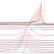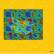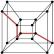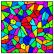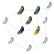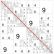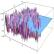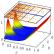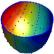## Contact

Coordinator: Prof. Dr. Stefan Müller